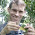## Friday, August 29, 2014

### Counting edges from a Newick string

I just posted a new phytools source build (phytools 0.4-32 featuring the newly updated `read.newick` function. `read.newick` is a simple tree-reading function - basically redundant with `read.tree` in the ape package, but slightly more tolerant of "badly conformed" Newick strings. Unfortunately, it is quite slow - although some improvements to the code have dramatically decreased run-time for the function.

At some point I will have to add these improvements to phytools `read.simmap` as it uses the same basic structure.

``````install.packages("phytools_0.4-32.tar.gz",type="source",repos=NULL)
``````
``````## Installing package into 'C:/Users/liam.revell/Documents/R/win-library/3.1'
## (as 'lib' is unspecified)
``````
``````library(phytools)
``````
``````## Loading required package: ape
``````

One of the tricks I employed to get `read.newick` to run faster was to figure out how many edges the tree should have before beginning to parse the Newick string. This turns out to involve simply counting the number of left parentheses (`")"`; or right parentheses as these will be the same) and the number of commas (`","`) and summing them. I'm a little embarassed to admit that the way I figured this out was by manually counting the number of commas and parentheses in several trees, setting up a system of linear equations, and solving for the coefficients; however in retrospect it is obvious: all edges in a tree written in Newick format (except for the root edge, which we actually don't care about anyway as it is a separate item in our `"phylo"` object anyway) have to be followed by either a `","` or a `")"`.

So, for instance:

``````trees<-allFurcTrees(n=5)
trees
``````
``````## 26 phylogenetic trees
``````
``````nedges<-sapply(trees,function(x) nrow(x\$edge))
nedges
``````
``````##   7 7 7 7 7 6 6 7 7 7 7 7 6 6 7 7 7 7 7 6 6 6 6 6 6 5
``````
``````## now let's create the Newick strings
obj<-write.tree(trees)
obj
``````
``````##   "(((3,4),2),1,5);" "(((3,2),4),1,5);" "((3,(4,2)),1,5);"
##   "((3,4),(1,2),5);" "((3,4),1,(5,2));" "((3,4),1,5,2);"
##   "((2,3,4),1,5);"   "((3,2),(1,4),5);" "(3,((1,4),2),5);"
##  "(3,((1,2),4),5);" "(3,(1,(4,2)),5);" "(3,(1,4),(5,2));"
##  "(3,(1,4),5,2);"   "(3,(2,1,4),5);"   "((3,2),1,(5,4));"
##  "(3,(1,2),(5,4));" "(3,1,((5,4),2));" "(3,1,((5,2),4));"
##  "(3,1,(5,(4,2)));" "(3,1,(5,4),2);"   "(3,1,(2,5,4));"
##  "((3,2),1,5,4);"   "(3,(1,2),5,4);"   "(3,1,(5,2),4);"
##  "(3,1,5,(4,2));"   "(3,1,5,4,2);"
``````
``````## count commas
ncommas<-sapply(obj,function(x) sum(strsplit(x,"")[]==","))
## count right brackets
nbrackets<-sapply(obj,function(x) sum(strsplit(x,"")[]==")"))
ncommas+nbrackets
``````
``````## (((3,4),2),1,5); (((3,2),4),1,5); ((3,(4,2)),1,5); ((3,4),(1,2),5);
##                7                7                7                7
## ((3,4),1,(5,2));   ((3,4),1,5,2);   ((2,3,4),1,5); ((3,2),(1,4),5);
##                7                6                6                7
## (3,((1,4),2),5); (3,((1,2),4),5); (3,(1,(4,2)),5); (3,(1,4),(5,2));
##                7                7                7                7
##   (3,(1,4),5,2);   (3,(2,1,4),5); ((3,2),1,(5,4)); (3,(1,2),(5,4));
##                6                6                7                7
## (3,1,((5,4),2)); (3,1,((5,2),4)); (3,1,(5,(4,2)));   (3,1,(5,4),2);
##                7                7                7                6
##   (3,1,(2,5,4));   ((3,2),1,5,4);   (3,(1,2),5,4);   (3,1,(5,2),4);
##                6                6                6                6
##   (3,1,5,(4,2));     (3,1,5,4,2);
##                6                5
``````
``````plot(nedges[order(nedges)],(ncommas+nbrackets)[order(nedges)],type="b")
``````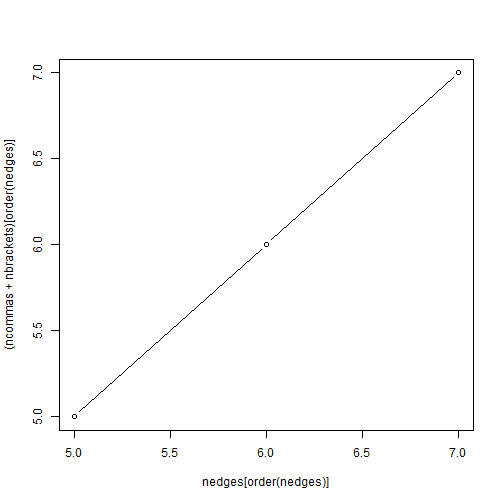This holds regardless of the tree size:

``````## n=6
trees<-allFurcTrees(n=6)
trees
``````
``````## 236 phylogenetic trees
``````
``````nedges<-sapply(trees,function(x) nrow(x\$edge))
obj<-write.tree(trees)
ncommas<-sapply(obj,function(x) sum(strsplit(x,"")[]==","))
nbrackets<-sapply(obj,function(x) sum(strsplit(x,"")[]==")"))
plot(nedges[order(nedges)],(ncommas+nbrackets)[order(nedges)],type="b")
``````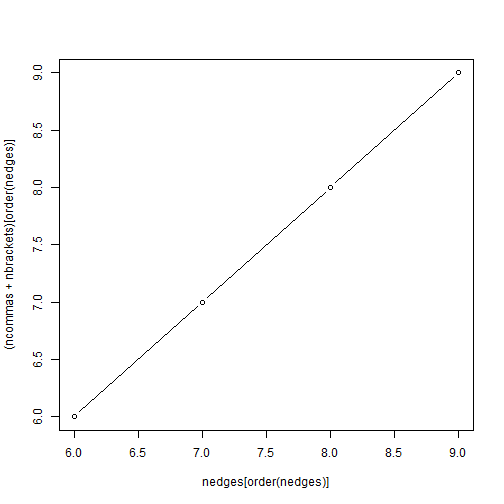``````## n=7
trees<-allFurcTrees(n=7)
trees
``````
``````## 2752 phylogenetic trees
``````
``````nedges<-sapply(trees,function(x) nrow(x\$edge))
obj<-write.tree(trees)
ncommas<-sapply(obj,function(x) sum(strsplit(x,"")[]==","))
nbrackets<-sapply(obj,function(x) sum(strsplit(x,"")[]==")"))
plot(nedges[order(nedges)],(ncommas+nbrackets)[order(nedges)],type="b")
``````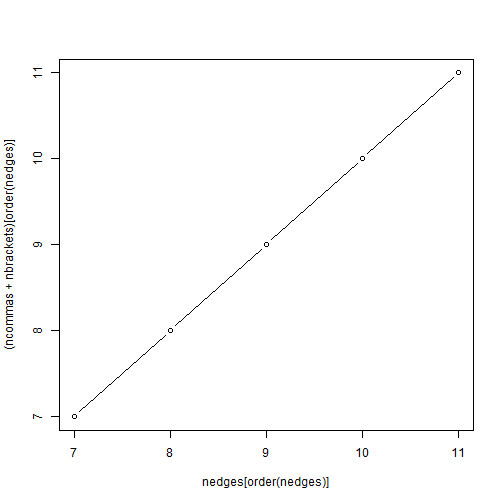``````## n=8
trees<-allFurcTrees(n=8)
trees
``````
``````## 39208 phylogenetic trees
``````
``````nedges<-sapply(trees,function(x) nrow(x\$edge))
obj<-write.tree(trees)
ncommas<-sapply(obj,function(x) sum(strsplit(x,"")[]==","))
nbrackets<-sapply(obj,function(x) sum(strsplit(x,"")[]==")"))
plot(nedges[order(nedges)],(ncommas+nbrackets)[order(nedges)],type="b")
``````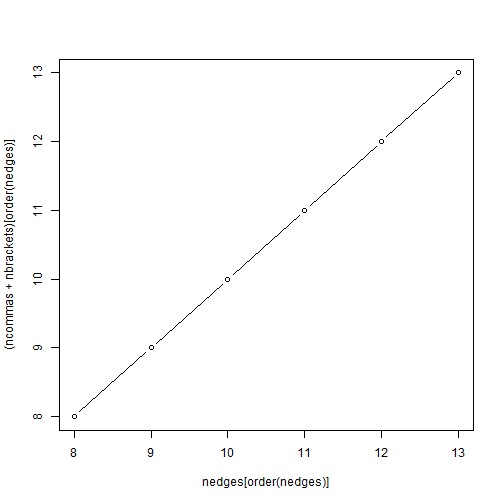1.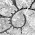1.